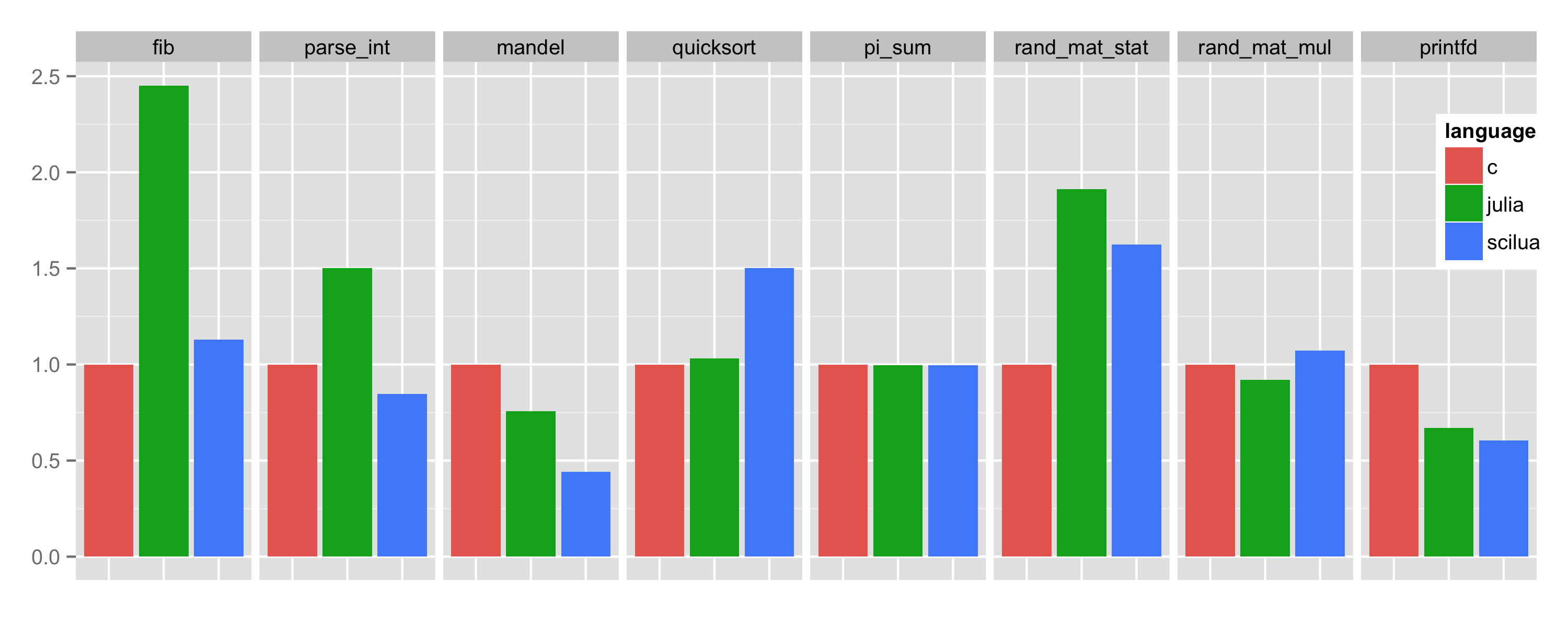# SciLua: Scientific Computing with LuaJIT

A complete framework for numerical computing based on LuaJIT which combines the ease of use of scripting languages (MATLAB, R, ...) with the high performance of compiled languages (C/C++, Fortran, ...).

Lua is a dynamic language characterized by a small number of simple but powerful concepts that allow to easily implement complex algorithms. Lua is fast to learn thanks to its high-level nature, to the concise syntax and to the precise documentation. Lua is a proven robust language that offers both object-oriented and functional programming features.

LuaJIT is an extremely optimized Just In Time compiler for Lua. Start-up and warm-up times are negligible, compile times are in the microsecond to millisecond range and the typical performance of compiled LuaJIT programs is close to the one of C/C++. LuaJIT includes the FFI library which allows to call C function directly.

## PerformanceThe figure above compares the running time required (lower is better) to run the Julia benchmark suite. Timings are relative to the C implementation.

The details: Linux x64 / gcc 5.2.1 / Julia 0.4.1 / SciLua 1.0 latest, implementation: perf-v1.2.sci.lua.

## Install

The easiest way to install SciLua is via the ULua distribution.

Having installed (i.e. unzipped) ULua, execute:


ulua/bin/upkg add sci        # Install the sci package (SciLua).
ulua/bin/upkg add sci-lang   # Install the sci-lang package (SciLua syntax extensions).


This is the suggested way as all dependencies are handled by the package manager and SciLua can be kept up to date using upkg update.

Alternatively, it is possible to install SciLua manually. Download and unzip sci~latest.zip and sci-lang~latest.zip into folders named respectively sci and sci-lang and make sure that such modules can be found by LuaJIT (check package.path documentation). Notice that SciLua requires xsys and OpenBLAS to be installed as well.

## Sci Library

The sci package contains a general purpose scientific computing library composed of the following modules:

Sub-ModuleDescription
sci.algvector and matrix algebra
sci.diffautomatic differentiation
sci.diststatistical distributions
sci.fminfunction minimization algorithms
sci.fmaxfunction maximization algorithms
sci.mathspecial mathematical functions
sci.mcmcMCMC algorithms
sci.prngpseudo random number generators
sci.qrngquasi random number generators
sci.quadquadrature algorithms
sci.rootroot-finding algorithms
sci.statstatistical functions

Each sub-module has to be loaded separately (require 'sci' is not allowed):


-- No global key is set:
local alg  = require "sci.alg"  -- Load sci.alg module.
local dist = require "sci.dist" -- Load sci.dist module.


## Sci Syntax Extensions

Based on the LuaJIT Language Toolkit, the sci-lang package contains the scilua executable which extends the syntax of LuaJIT for easier linear algebra operations:

• algebra expressions constructed via empty bracket [] indexing
• element-wise operations via plain Lua operators +-*/^%
• matrix multiplication via **
• matrix exponentiation via ^^
• transposition via 
• efficient implementation minimizes required allocations and loops
• support for assignments

For example, the rand_mat_stat benchmark shown above is implemented as:


local function randmatstat(t)
local n = 5
local v, w = alg.vec(t), alg.vec(t)
for i=1,t do
local a, b, c, d = randn(n, n), randn(n, n), randn(n, n), randn(n, n)
local P = alg.join(a..b..c..d)
local Q = alg.join(a..b, c..d)
v[i] = alg.trace((P[]**P[])^^4) -- Matrix transpose, product and power.
w[i] = alg.trace((Q[]**Q[])^^4) -- Matrix transpose, product and power.
end
return sqrt(stat.var(v))/stat.mean(v), sqrt(stat.var(w))/stat.mean(w)
end
`